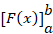-->

# Calculate the area of ​​an object using the integral method

A problem that we often face is if we are faced with the calculation of the building area that is limited not by a straight line. An example is how we calculate the area of ​​a wake in Figure 1 below,

To find the building area approach is to make plots in the form of a unit square as shown in Figure 1. If we consider the area of ​​the inner and outer areas of the building, the building area is between 12 and 16 area units.

Furthermore, the area of ​​a closed curve in the Cartesian plane will be studied. Figure 2 shows an area limited by the curve y = f(x), X axis, line x = a, and x = b.

To obtain a broad approach to the area, the method is to make several vertical lines (strips) such that they form a rectangle. The total area of ​​the rectangle is the area wide approach.
Suppose that one of the rectangular pieces is named PP‘Q’Q with the coordinates of point P (x, y). The rectangle width P'Q' we call akanx, and the area of ​​PP‘Q’Q we call δL. The area of ​​a rectangle is the length of the width, then δL = y δx

The number of rectangular areas from x = a to x = b can be expressed with
With n is a lot of rectangles. The total area calculation will be accurate if the selected δx is very small to near zero, that is, in a limit. So,

We can write the form above as an integral form below,

Our next question is whether the area in question is an anti-derivative of a function? To answer these questions, will be explained in the next chapter.

Example 1.
Show with an area shading that is formulated with an integral form, below

First draw each curve whose equation is y = 2x + 3 and y = x2, then make the shading as mentioned above.

### Fundamental Theorem

Theorem: If f is a continuous function in the intervals [a, b] and F is anti derivative f on [a, b], then

Proof of the function f (x) in Intergral Fundamental is as follows,

The shaded area in Figure 5 is the area bounded by the curve y = f (x), X axis, line x = a, and x = b. Suppose the rectangle width in the image is h, then the area of ​​PP1Q1Q is

L(x + h) = L(x)
And this area of ​​magnitude lies between the area of ​​a small rectangle and a large rectangle, then
For h à 0 then,

The area of ​​x = a to x = b is

### Certain Intergral

The form of writing F(b) - F(a) on the Fundamental Theorem is usually expressed by. Writing in full is as follows:
Note: The integral written in the notationis called the Specific Integral because the result is a certain value, a is called the lower limit and b is called the integral upper limit.

Example 2.